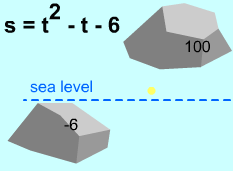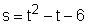Ch 1. Limits Multimedia Engineering Math Limit of aSequence Limit of aFunction LimitLaws Continuity RateChange
 Chapter 1. Limits 2. Derivatives I 3. Derivatives II 4. Mean Value 5. Curve Sketching 6. Integrals 7. Inverse Functions 8. Integration Tech. 9. Integrate App. 10. Parametric Eqs. 11. Polar Coord. 12. Series Appendix Basic Math Units Search eBooks Dynamics Fluids Math Mechanics Statics Thermodynamics Author(s): Hengzhong Wen Chean Chin Ngo Meirong Huang Kurt Gramoll ©Kurt GramollMATHEMATICS - CASE STUDY IntroductionMoving Process Jim is surveying the height of two rocks in Death Valley. After the measurement, he finds that one is 6 feet below sea level, and the other is about 100 feet above sea level. When he moves from the first rock to the second, he notices that elevation, s, is a function of time: s = t2 - t - 6. Jim wondered how many times he passed through the sea level during his travel. The distance unit is feet and the time unit is minute. What is known: The elevation function is:One rock is 6 feet below sea level, the other rock is 100 feet above sea level. Questions Does Jim pass through sea level? If yes, how many times and when will he pass through it? Approach Use the corollary of intermediate value theorem.